# PSEB 9th Class Science Important Questions Chapter 4 Structure of the Atom

Punjab State Board PSEB 9th Class Science Important Questions Chapter 4 Structure of the Atom Important Questions and Answers.

## PSEB 9th Class Science Important Questions Chapter 4 Structure of the Atom

Question 1.
What is Rutherford’s ∝-ray scattering experiment? Give its observations. How does Rutherford explain this observation?
Rutherford’s ∝-ray scattering experiment: In this experiment, Rutherford obtained a thin stream of fast-moving alpha (∝) particles from radioactive source (P0) and was allowed to strike against a thin foil of heavy metal like gold. Alpha particles are also called helium nuclei (He2+ with + 2 unit charge and 4 a.m.u. mass). As a result of the experiment, he made the following observations:

1. Most of the ∝-particles passed through the gold foil without any deflection from their original path.
2. Some of the ∝-particles got deflected by small angles from their original path.
3. A few ∝-particles (approximately 1 out of 10,000) were deflected to a large extent. In some cases, the a-particles came back in the same direction after striking the gold foil.

Explanation for the observations:

We know that the gold foil is made up of atoms of gold which are placed side by side. Since most of the ∝-particles passed through these atoms undeflected they did not come across any obstruction in their path, this shows that most of the space in the atom is light or hollow.

The electrons with negligible mass were supposed to be present in this portion called extra nuclear portion. The a-particies were deflected from their path and a very few came back in the same direction. This shows that they must have met with some obstruction in their path.
The obstruction must be
1. Heavy because a-particles are high speed particles and can remove light obstruction from their path.
2. Small because only a few a-particles got deflected.
3. Positively charged because some a-particles were repelled by this obstruction.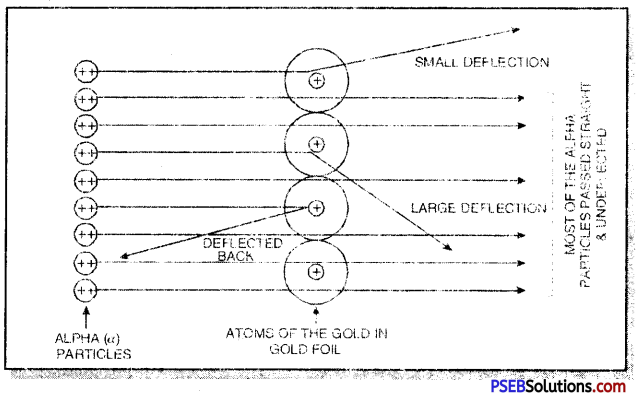This small, heavy and positively charged portion inside an atom was called nucleus. The protons with mass and positive charge were supposed to be present in the nucleus. Later on, neutrons were also found to be present in the nucleus of the atom.Question 2.
Discuss in brief Rutherford’s Model of atom.
The main points of Rutherford’s model of atom are listed as follows:
1. An atom has two parts. These are nucleus and extra nuclear portion.
2. The nucleus contains protons while electrons are present in the extra nuclear portion.
3. Most of the mass of the atom is due to the nucleus because electrons have negligible mass.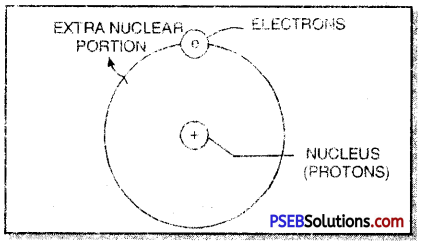4. The volume occupied by the nucleus is very small as compared to the volume occupied by extra nuclear portion.
5. The electrons in the extra nuclear portion are not stationary. These are revolving around the nucleus. The electrons are called planetary electrons because they can be compared to various planets which revolve around the sun. The nucleus may be compared with the sun.

Question 3.
Discuss the arrangement of the electrons in the different energy levels.
Or
Describe Bohr-Bury scheme for the distribution of electrons in the various orbits or energy levels of an atom.
The distribution of electrons in the energy levels is given with the help of Bohr Bury scheme. It is given as follows:
1. The energy levels or energy shells are filled in order of increasing energies. The electrons first enter the K shell (n = 1. which is closest to the nucleus. This is followed by L shell (n = 2), M shell (n = 3) and so on. Here V represents the number of the shell.
2. The maximum number of electrons in any shell is given as 2n2 (n = number of the shell). The distribution in the first four energy shells is given in the table given below:
Distribution of electrons in first four energy shells according to 2n2 rule.

 Symbol of shell Number of shell (n) Maximum number of electrons (2n2) K 1 2 x (1)2 = 2 L 2 2 x (2)2 = 8 M 3 2 x (3)2 = 18 N 4 2 x (4)2 = 32

3. The outermost energy shell cannot have more than 8 electrons. The next inner shell called penultimate shell, cannot have more than 18 electrons.
4. It is not necessary that an energy level or shell is fully filled before the filling in the next energy level starts. In fact, filling of electrons in a new shell starts when any shell contains 8 electrons.
On the basis of the above scheme, let us discus with mass number 24 and atomic number 12.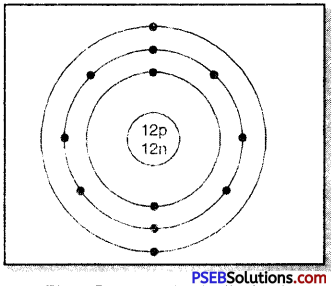• Mass no. of atom (A) = 24
• Atomic no. of atom (Z) = 12
• No. of protons in nucleus = 12
• No. of neutrons in nucleus = 12
• No. of electrons to be distributed = 12
• The distribution in the various energy shells: K L M: 2, 8, 2Question 4.
Give the electronic distribution in the first twenty elements on the basis of Bohr-Bury scheme.
On the basis of Bohr-Bury scheme, the distribution of electrons in first twenty elements (Z = 1 to Z = 20) is given below:
Table: Electronic distribution in atoms of first 20 elements

 Element (Symbol) Mass No. (A) Atomic No. (Z) No. of Protons (P) No. of Electrons (e) No. of Neutrons (n) (A-Z) Electronic Distribution K L M N 1. Hydrogen (H) 1 1 1 1 0 1 2. Helium (He) 4 2 2 2 2 2 3. Lithium (Li) 7 3 3 3 4 9 1 4. Beryllium (Be) 9 4 4 4 5 2 2 5. Boron (B) 11 5 5 5 6 2 3 6. Carbon (C) 12 6 6 6 6 2 4 7. Nitrogen (N) 14 7 7 7 7 2 5 8. Oxygen (O) 16 8 8 8 8 2 6 9. Fluorine (F) 19 9 9 9 10 2 7 10. Neon (Ne) 20 10 10 10 10 2 8 11. Sodium (Na) 23 11 11 11 12 2 8 1 12. Magnesium (Mg) 24 12 12 12 12 9 8 2 13. Aluminium (Al) 27 13 13 13 14 ‘ 2 8 3 14. Silicon (Si) 28 14 14 14 14 2 8 4 15. Phosphorus (P) 31 15 15 15 16 2 8 5 16. Sulphur (S) 32 16 16 16 16 2 8 6 17. Chlorine (Cl) 35 17 17 17 18 2 8 7 18. Argon (Ar) 40 18 18 18 22 2 8 8 19. Potassium (K) 39 19 19 19 20 2 8 8 1 20. Calcium (Ca) 40 20 20 20 20 2 8 8 2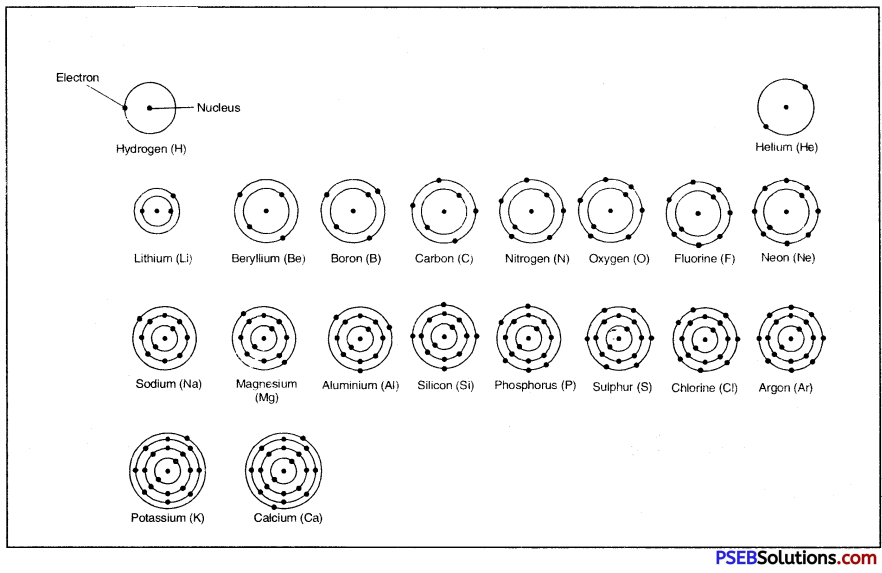Special cases of Potassium and Calcium:

• In case of Potassium (Z = 19), the M shell has only 8 electrons and not 18. Actually, when the M shell gets 8 electrons, the filling of electrons starts in the next shell which is N-shell. When the N-shell gets 2 electrons, the electron are again- filled in the M shell.
• Thus, electronic distribution in potassium (Z = 19) is
K L M N
2 8 8 1 (M shell has 8 electrons and N shell has 1 electron)
• Similarly, the electronic distribution in calcium (Z = 20) is:
K L M N: 2 8 8 2 (M shell has 8 electrons and N shell has 2 electrons)Question 5.
Define the terms:

1. Atomic number
2. Mass number
3. Atom
4. Ion
5. Element
6. Orbit
7. Nucleons

1. Atomic number: It is the number of protons present in the nucleus of an atom of the element.
2. Mass number: It is the sum of number of protons and neutrons present in the nucleus of an atom of the elements.
3. Atom: It is the smallest unit of matter which takes part in chemical reactions and it may or may not exist independently.
4. Ion: It is an atom or group of atoms having charge positive or negative and can exist freely in solution.
5. Element: It is a pure substance which can neither be built up nor broken into two or more still simpler substance by any physical or chemical method and it consists of only one kind of atoms.
6. Orbit: It is the fixed circular path traced by an electron revolving around the
7. Nucleons: The protons and neutrons present in the nucleus of an atom of the element are collectively known as nucleons.u

Question 1.
What do you understand by the structure of atom?
According to the Dalton’s atomic theory, the smallest particle of matter is called atom. But later studies have shown that an atom is further made up of sub-atomic particles called electrons, protons and neutrons. Different atoms have different number of such particles and they also differ in the arrangement of these particles. Therefore, the atoms have different properties.

Question 2.
What happens when electric field is applied to the path of cathode rays? What does it show?
When electric field is applied to the path of the cathode rays, these are deflected towards the positive plate of the field. This shows that the cathode rays consist of negatively charged particles.Question 3.
What is the information conveyed by the following observations :

1. Atom is electrically neutral,
2. Mass of the atom is due to the nucleus.

1. If an atom is electrically neutral, this means that it will have equal number of protons and electrons. Both protons and electrons have 1 unit charge but with opposite sign.
2. This means that nucleus contains in it both protons and neutrons which have mass.

Question 4.
What is the significance of number of protons found in the atoms in each of the different elements?
The number of electrons in atom of the element is equal to the number of protons. By knowing no. of protons, we can calculate no. of electrons and hence its properties.

Question 5.
Write the electronic configuration of the elements A, B, C, D, E with atomic numbers 5, 6, 14, 13 and 15. Which have similar chemical properties?

 Element Atomic No. (Z) Electronic configuration K L M A 5 2 3 B 6 2 4 C 14 2 8 4 D 13 ? 8 3 E 15 2 8 5

Elements B and C. have similar chemical properties because both of them have 4 valence elt, trons.
Elements A and D have similar chemical properties because both of them have 3 valence electrons.

Question 6.
Five species P, Q, R, S, T have electrons, protons and neutrons are as follows:

 Species Electrons Protons Neutrons P 4 3 4 Q 8 9 9 R 17 17 20 S 17 17 18 T 18 18 22

Find

1. a cation
2. an anion
3. a noble gas and
4. a pair of isotopes.

1. Cation – Q
2. Anion – P
3. A noble gas = T
4. Pair of isotopes = R and S (because they have same number of electrons and protons but different number of neutrons).Question 7.
Helium has only 2 electrons in the K-shell. But it is called an inert gas element. Why?
Helium (He) atom has only one shell (K-shell) which can have a maximum of 2 electrons only. It cannot have more than 2 electrons. As a result, the combining capacity of He is zero. Therefore, its valency is also zero.

Question 8.
Give labelled diagram to show how are cathode rays produced?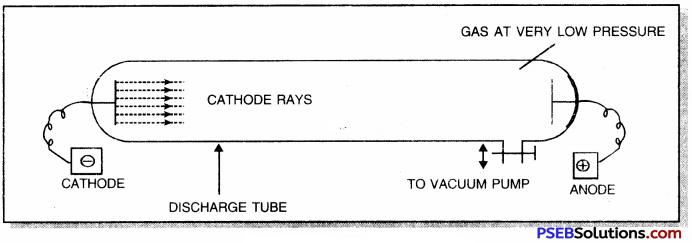Question 9.
An atom has mass number A and atomic number Z.
1. How many protons are present in the nucleus?
2. How many electrons are revolving around the nucleus?
3. How many neutrons are present in its nucleus?
Mass number = A
Atomic number = Z
1. No. of protons present in the nucleus = Z
2. No. of electrons revolving around the nucleus = Z
3. No. of neutrons present in the nucleus = A – Z.

Question 10.
State three ways by which a proton differs from an electron.

 Proton Electron 1. It has one unit of positive charge. 2. It has mass 1.6 x 10-24 g. 3. It is present in the nucleus of an atom. It has one unit of negative charge. It has mass 9.1 x 10″28 g. It is present in the extranuclear part of an atom.

Question 11.
What are isotopes? Give two uses of isotopes. Name the isotopes of hydrogen. Give their structures.
Isotopes. The atoms of the same element having the same atomic number but different mass numbers are called isotopes.
Use of isotopes:
1. Isotopes of hydrogen, oxygen, carbon are used to study the mechanisms of organic reactions.
2. Some radioactive isotopes are used for the treatment of cancer and other diseases. The isotopes of hydrogen are: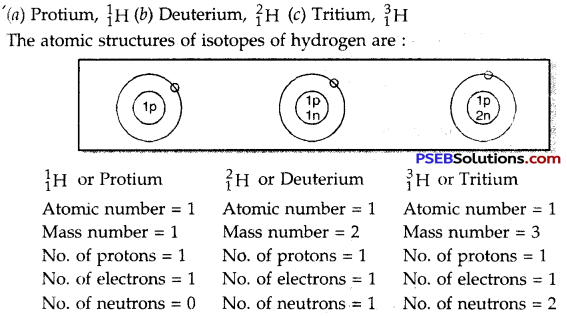Question 12.
Explain why elements have fractional atomic masses?
The elements have fractional atomic masses due to the existence of isotopes (having different mass numbers) e.g. chlorine has two isotopes $${ }_{17}^{35} \mathrm{Cl}$$ and $${ }_{17}^{37} \mathrm{Cl}$$ and are present in the ratio 3:1.
∴ Average atomic mass of chlorine = $$\frac{35 \times 3+37 \times 1}{4}=\frac{105+37}{4}$$ = 35.5 a.m.u.

Question 13.
What are isobars? Give examples.
The atom of different elements having same mass numbers but different atomic numbers are called isobars.
e.g. 1. $${ }_{18}^{40} \mathrm{Ar}$$ and $${ }_{19}^{40} \mathrm{Ar}$$
2. $${ }_{36}^{86} \mathrm{Ar}$$ and $${ }_{38}^{86} \mathrm{Ar}$$

Question 14.
Sulphur has an atomic number 16 and a mass of 32. State the number of protons and neutrons in the nucleus of sulphur. Give a simple diagram to show the arrangement of electrons in an atom of sulphur.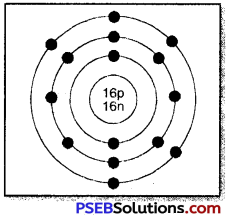Atomic number of S = 16
Mass number of S = 32
∴ No. of protons = 16
No. of neutrons = 32 – 16 = 16
No. of electrons = 16

Question 15.
Write down the electronic configuration of the following:
1. $${ }_{13}^{27} \mathrm{X}$$
2. $${ }_{17}^{35} \mathrm{X}$$
Write down the number of electrons in X and neutrons in Y and the formula of
compound formed by X and Y.
1. $${ }_{13}^{27} \mathrm{X}$$
Atomic number of X = 13
Mass number of X =27
No. of protons in X = 13
∴ No. of electrons in X = 13
The electronic configuration of X = 2, 8, 3
Valency of X = 3

2. $${ }_{17}^{35} \mathrm{X}$$
Atomic number of Y = 17
Mass number of Y =35
No. of protons in Y = 17
No. of electrons in Y = 17
No. of neutrons in Y = 35 – 17 = 18
The electronic co0nfiguration of Y = 2, 8, 7
Valency of Y = 1
The formula of the compound formed by X and Y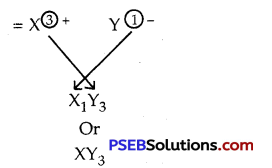Question 16.
Give the important properties or characteristics of isotopes.

1. The isotopes of an element have same atomic number (Z) i.e. the same number of electrons and same number of protons. Hence, they have identical chemical properties.
2. Isotopes of an element have same number of valence electrons and hence same valency.
3. Isotopes of an element have different mass numbers (or different number of neutrons) and hence they have different physical properties such as mass, density, melting point, boiling point etc.

Question 17.
An atom of the element is represented as 11x?

1. What does the numeral 23 indicate?
2. What does the nemeral 11 indicate?
3. What is the number of protons in X?

1. 23 indicates mass number of X.
2. 11 indicates atomic number of X.
3. No. of protons in X = Atomic number = 11.

Question 18.
The atom of an element is made up of 4 protons, 5 neutrons and 4 electrons. what are its atomic number and mass number?
No. of proton = 4
Atomic number of the element, Z = 4
No. of neutrons = 5
∴ No. of protons + No. of neutrons =4 + 5 = 9
∴ Mass number of the element, A = 9.

Question 19.
Calculate the number of neutrons in the following elements:
1. $${ }_{1}^{3} \mathrm{Cl}$$
2. $${ }_{17}^{37} \mathrm{Cl}$$
3. $${ }_{20}^{40} \mathrm{Cl}$$
1. $${ }_{1}^{3} \mathrm{Cl}$$
Mass number = 3
Atomic number = 1
No.of neutrons = Mass no – Atomic no.
= 3 – 1 = 2

2. $${ }_{17}^{37} \mathrm{Cl}$$
Mass number = 37
Atomic number = 17
No.of neutrons = 37 – 17 = 20

3. $${ }_{20}^{40} \mathrm{Cl}$$
Mass number = 40
Atomic number = 20
No.of neutrons = 40 – 20 = 20

Question 20.
Give two conditions under which cathode rays are produced.
Cathode rays are produced when a gas is subjected to the action of
1. High voltage of the order of 5000-10000 V and
2. Under a low pressure of the order of 10-3 atm of Hg.Question 21.
The electronic configuration of P (Z = 15) is 2, 8, 5. Why is the valency of phosphorus 3 and not 5?
The electronic configuration of phosphorus (P) shows that it has 5 valence electrons. Its valency is expected to be 5. But it is not possible for the atom to lose 5 electrons present in the valence shell because the energy required to remove these electrons will be very high. Therefore, phosphorus atom has valency equal to 3 because to gain 3 electrons is easier than losing 5 electrons as it requires less energy.

Question 22.
What information is conveyed by the statement that the mass number of magnesium is 24 and the atomic number is 12?
The atomic number (12) of magnesium indicates that its atom has 12 protons in the nucleus and an equal number of electrons in the extra nuclear portion. The mass number (24) points out that there are also 12 neutrons (24 – 12 = 12) present in the nucleus alongwith protons.

Question 23.
Is it possible for an atom to have 12 protons and 13 electrons? Explain.
No, it is not possible. An atom must always be electrically neutral. This means that it has no net charge present on it. Now, each proton has one unit positive charge and each electron has one unit negative charge. In a neutral atom, the number of electrons and protons must always be the same. Thus, an atom cannot have 12 protons and 13 electrons.

Question 24.
Give the important isotopes of hydrogen, carbon, oxygen and chlorine. Indicate their mass numbers and atomic numbers.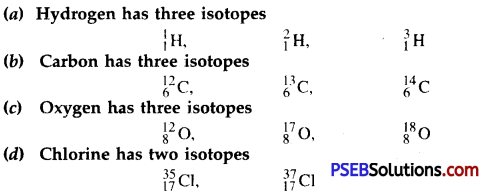Numerical Problems (Solved):

Question 1.
Chlorine has two isotopes $${ }_{17}^{35} \mathrm{Cl}$$ and $${ }_{17}^{37} \mathrm{Cl}$$ in the ratio 3 : 1. Calculate the average atomic mass of chlorine.
Solution: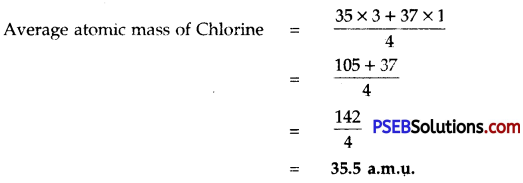Question 2.
Out of 18X and 16Y which atom is chemically more reactive?
Solution: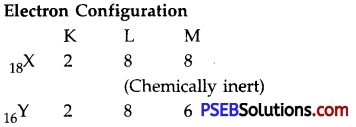No. of valence electrons in X =8
No. of valence electrons in Y =6
∴ Y is more reactive.

Question 3.
An element has 12 neutrons and mass number 23. Give the atomic number and symbol of the element.
Solution:

• Mass number(A) = 23
• Number of neutrons = 12
• Number of protons = 23 – 12 = 11
• Atomic Number(Z)= 11
• Symbol of the element = $$\frac{23}{11} \mathrm{Na}$$

Question 4.
Calculate number of protons, neutrons and electrons in $$\frac{235}{92} \mathrm{U}$$ and $$\frac{238}{92} \mathrm{U}$$. How are these atoms related?
Solution:
table
$$\frac{235}{92} \mathrm{U}$$ and $$\frac{238}{92} \mathrm{U}$$ are isotopes.

Question 5.
Calculate the number of electrons, protons and neutrons is $$\frac{19}{9} \mathrm{U}$$. Also calculate its valency.
Solution:

1. Atomic number = 9
2. Mass number = 19
3. No. of protons = 9
4. No. of neutrons = 19 – 9 = 10
5. Electronic configuration = 2 7
6. Valency = 8 – 7 = 1.

Question 1.
Who discovered canal rays?
Goldstein discovered canal rays in 1886.Question 2.
Which charge is present on canal ray particles?
They carry positive charge.

Question 3.
Which charge is present on protons?
Positive charge.

Question 4.
Where are protons present in an atom?
Protons are present in the nucleus, deep inside the atom.

Question 5.
What were the characteristics of an atom according to Dalton?
According to Dalton an atom was indivisible and indestructible.

Question 6.
Which discovery is against Dalton’s theory?
Discovering of electrons and protons in an atom.

Question 7.
Who proposed the first model for the structure of atom?
J.J. Thomson.Question 8.
Why did Rutherford used gold foil in a-ray scattering experiment?
This is because he wanted as thin a layer as possible. It was about 1000 atoms thick.

Question 9.
What are α-particles?
They are doubly charged helium ions (He2+).

Question 10.
What is the charge on α-particles?
+2 units.

Question 11.
What is the mass of an α-particle?
4 u.

Question 12.
How the defects of Rutherford’s model were removed by Neil Bohr?
The electrons revolve around the nucleus in an atom in fixed circular paths called orbits or energy levels and don’t lose energy.

Question 13.
What are energy levels or shells?
The definite orbits in where electrons revolve around the nucleus are called energy levels. These are designated as K, L, M, N ……………. 1, 2, 3, 4.Question 14.
Define mass number of an element.
It is the sum of number of neutrons and protons present in the nucleus of the atom of the element.

Question 15.
Who gave the distribution of electrons in the various shells of an atom?
Bohr and Bury.

Question 16.
What is the maximum number of electrons present in an electronic shell?
2n2 (Where n is the number of shells).

Question 17.
What is the maximum first four shells of an atom?
2, 8, 18, 32.

Question 18.
What is the maximum number of electrons in the outermost shell of an atom?
8

Question 19.
Which elements are chemically inert?
These are the elements which have 8 electrons in their outermost shells.Question 20.
Define octet.
The maximum number of electrons present in the outermost shell of an atom is called octet.

Question 21.
Define valency of an element.
It is the combining capacity of an atom of the element and it is equal to the number of electrons lost or gained or contributed for sharing from its valence shell in order to complete its octet.

Question 22.
Define atomic number.
It-is the number of protons present in the nucleus of an atom of the element.

Question 23.
Define nucleon.
The neutrons and protons present in the nucleus of an atom of the element are collectively known as nucleons.

Question 24.
Where is most of the mass of the element present?
In the nucleus of an atom.

Question 25.
Define atomic mass of an element.
It is the sum of the number of neutrons and protons present in the nucleus of its atom.

Question 26.
What are isotopes?
Atoms of the same element having same atomic number but different mass numbers are called isotopes.Question 27.
Write two isotopes of chlorine.
$${ }_{17}^{35} \mathrm{Cl}$$ and $${ }_{17}^{37} \mathrm{Cl}$$.

Question 28.
Write two isotopes of carbon.
$${ }_{6}^{12} \mathrm{Cl}$$ and $${ }_{6}^{14} \mathrm{Cl}$$.

Question 29.
Isotope of which element is used in the treatment of cancer?
Isotope of cobalt ($${ }_{27}^{59} \mathrm{Co}$$).

Question 30.
What is the atomic mass of chlorine?
35.5

Question 31.
Isotope of which element is used for the treatment of goitre?
An isotope of iodine.

Question 32.
Isotope of which element is used in atomic reactor?
Uranium.

Question 33.
What are isobars?
Atoms of the different elements having same mass number but different atomic numbers are called isobars.

Question 34.
Give one example of isobars?
$$\underset{(\text { Calcium })}{\stackrel{40}{20} \mathrm{Ca}}$$ and $$\underset{(\text { Argon })}{\stackrel{40}{18} \mathrm{Ca}}$$.

Question 35.
Write three isotopes of hydrogen.

1. Protium ($${ }_{1}^{1} \mathrm{H}$$)
2. Deuterium ($${ }_{2}^{1} \mathrm{H}$$)
3. Tritium ($${ }_{3}^{1} \mathrm{H}$$).Question 36.
What is the total number of electrons in an atom if its K-and L-shells are fully filled?
10 (K-2, L-8).

Question 37.
What electrons in an atom influence its chemical properties?
Valence electrons (electrons in the outermost shell).

Question 38.
How will you represent chlorine atom having mass number 35 and atomic number 17?
$${ }_{17}^{35} \mathrm{Cl}$$

Question 39.
Name the sub-atomic particle on which size of an atom depends?
Electron.

Question 40.
Name the atoms having same atomic number but different mass numbers.
Isotopes.Question 41.
Mg2+ has 10 electrons, what is the number of protons in it?
10 + 2 = 12.

Question 42.
Name the element which has no neutrons in the nucleus of its atom.
Hydrogen.

Question 43.
Out of electrons, protons and neutrons, which are same in isotopes?
Electrons and protons.

Question 44.
There are three isotopes of hydrogen, $${ }_{1}^{1} \mathrm{H},{ }_{1}^{2} \mathrm{H},{ }_{1}^{3} \mathrm{H}$$ all are electrically neutral, why?
This is because each isotope has one electron and one proton.

Question 45.
An element has atomic number 16. Give its electronic configuration and number of valence electrons.
Electronic configuration 2, 8, 6.

Question 46.
The number of electrons in the valence shell of sodium (Na) is ……………… .
1.

Question 47.
Fluorine belongs to …………… family.
Halogen.Question 48.
In an atom, the number of …………… is the same as the number of ………………. .
Electrons, protons

Question 49.
Sodium has …………….. electron …………….. than sodium ion.